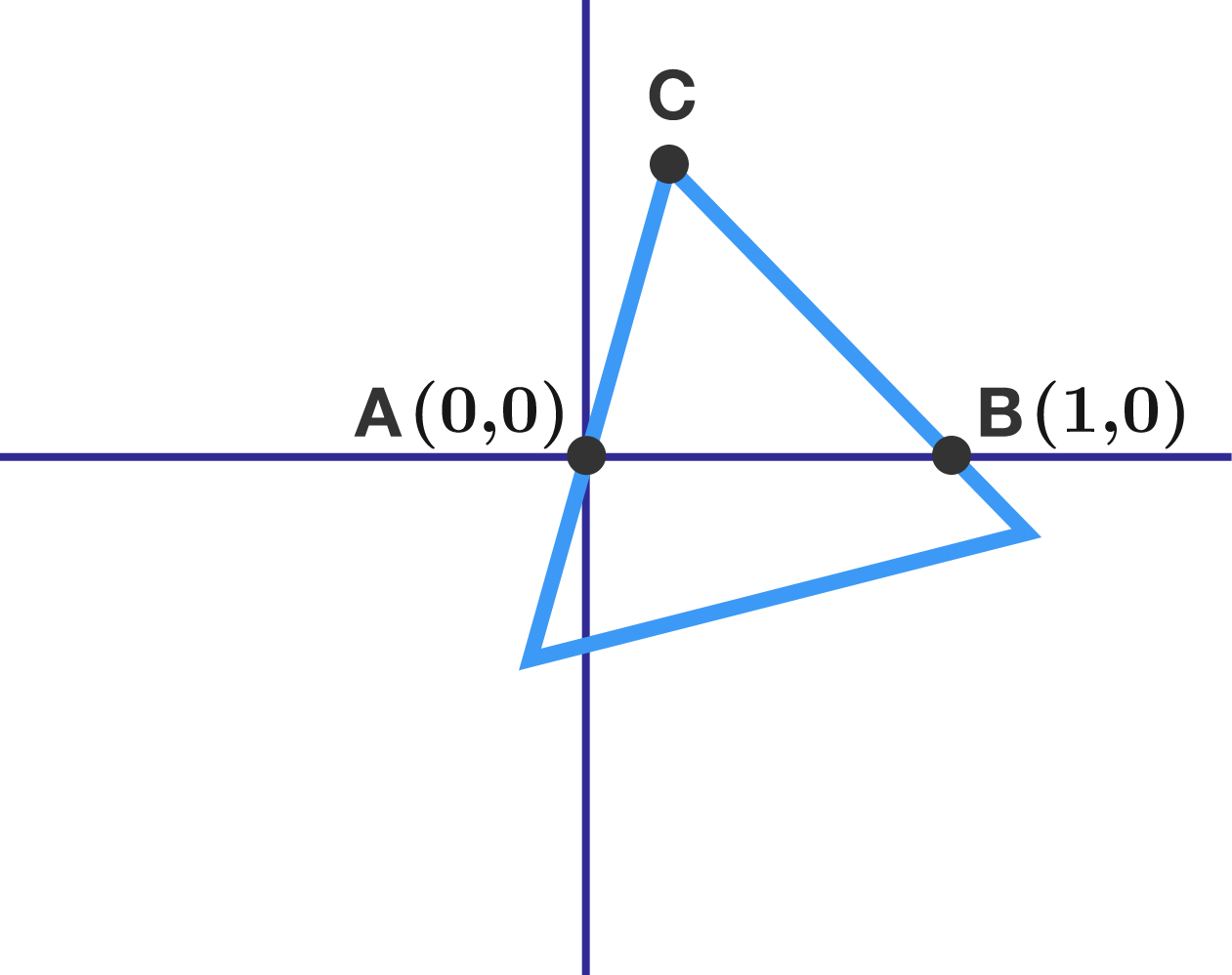# An equilateral triangle

Geometry Level 4An equilateral triangle

• has two of its vertices below the $x$-axis,
• has the third vertex $C$ above the $x$-axis, and
• contains $A=(0,0)$ and $B=(1,0)$ on its sides.

How long is the path traced out by all possible points $C,$ to two decimal places?

If you think the path is infinitely long, provide 99999 as your answer. Or, if you think all the possible locations of $C$ represent an area rather than a path, submit 99998 as your answer.

×